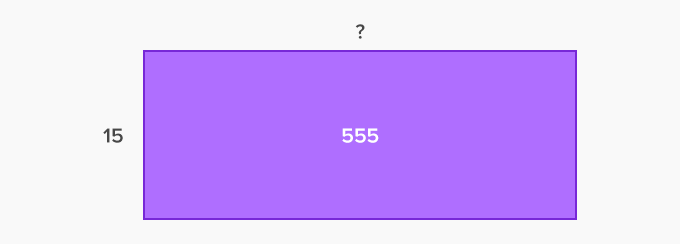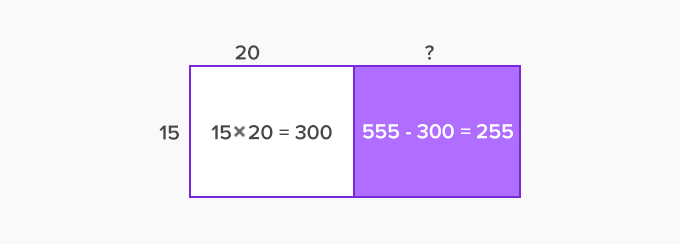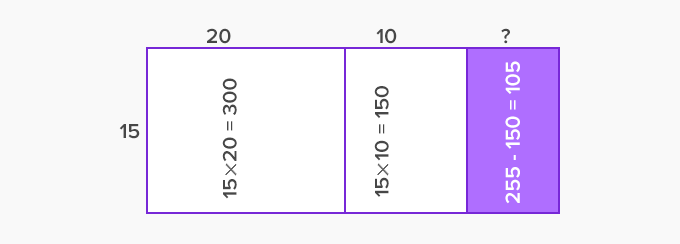# Area Model Division - Definition with Examples

The Complete K-5 Math Learning Program Built for Your Child

• 40 Million Kids

Loved by kids and parent worldwide

• 50,000 Schools

Trusted by teachers across schools

• Comprehensive Curriculum

Aligned to Common Core

## What is Area Model Division?

The area of a shape is the space occupied by the shape

If a rectangle has a length equal to 32 units and a width of 23 units, then we can calculate this area by taking the product 32 ⨉ 23. In other words, when we consider the product 32 ⨉ 23, geometrically it can be interpreted as the area of a rectangle of the length of 32 units and width 23 units.

Similarly, we can geometrically interpret a division problem, say, 555 ÷ 15, as the missing dimension of a rectangle of area 555 sq. units and one side 15 units long.

• The rectangle can be further divided into smaller rectangles, repeatedly calculating the length of each smaller rectangle. These lengths can be added to get the required length.• First, consider a smaller rectangle of the height of 15 units and a length of 20 units. So, the area of the rectangle would be 300 square units, and the rest of the rectangle has an area of 555 – 300 or 255 sq. unit.Now, we have an area of 255 sq. units for sub-division. Since 15⨉10 = 150, another rectangle of height 15 units and length 10 units can be marked.Finally, we have 15 ⨉ 7 = 105. So, the shaded rectangle above has a height of 15 units and length 7 units constituting an area of 105 sq. units.

Thus, the length of the rectangle is 20 + 10 + 7 units or 37 units. Therefore, 555 ÷ 15 = 37.

Example: Catherine has 540 saplings to plant in her farm. If she can plant 30 of them in a row, how many such rows of saplings would be there?

The number of rows is given by the quotient 540 ÷ 30. Now, in the area model division, we need to find the missing dimension of a rectangle that has an area of 540 sq. units and length of 30 units.

Step 1:Step 2:Thus, 540 ÷ 30 = 18. Therefore, 18 rows of saplings would be there.

 Fun Facts If the difference is greater than the divisor, the division is incomplete! If the difference is less than the divisor, then it is the REMAINDER! Can you start the procedure with another length of the rectangle? Yes, for example, in 555 ÷ 15, if we consider the sub-rectangle of length 5 units then the area becomes 15 ⨉ 5 or 75 sq. units and the rest of the area become 555 − 75 = 480 sq. units. The procedure can be continued in a similar way.

Won Numerous Awards & Honors